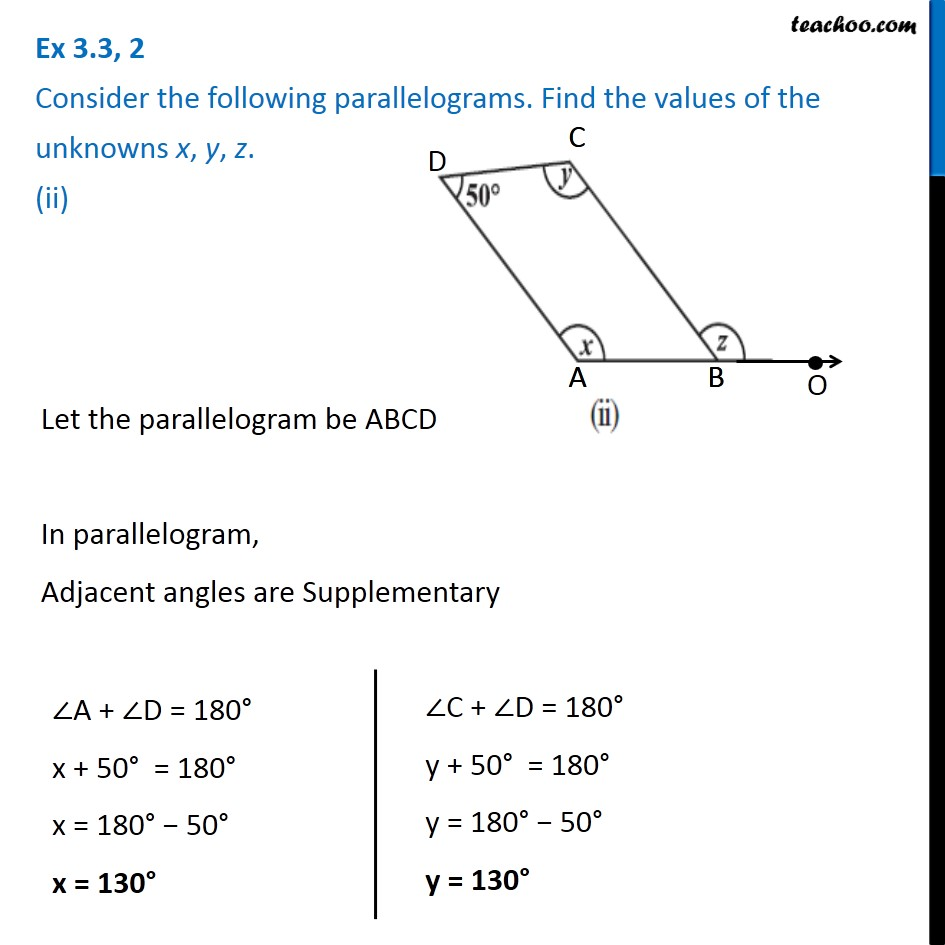Ex 3.3

Chapter 3 Class 8 Understanding Quadrilaterals
Serial order wiseLearn in your speed, with individual attention - Teachoo Maths 1-on-1 Class

### Transcript

Ex 3.3, 2 Consider the following parallelograms. Find the values of the unknowns x, y, z. (ii) Let the parallelogram be ABCD In parallelogram, Adjacent angles are Supplementary ∠A + ∠D = 180° x + 50° = 180° x = 180° − 50° x = 130° ∠C + ∠D = 180° y + 50° = 180° y = 180° − 50° y = 130° Also, Opposite angles of a Parallelogram are equal ∠ABC = ∠ D ∠ ABC = 50° Now, ABO is a line So, by linear pair ∠ ABC + z = 180° 50° + z = 180° z = 180° − 50° z = 130° Therefore, x = 130° , y = 130° and z = 130°